Next: Worked example 8.4: Weight Up: Rotational motion Previous: Worked example 8.2: Accelerating

## Worked example 8.3: Moment of inertia of a rod

Question: A rod of mass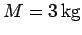and length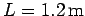pivots about an axis, perpendicular to its length, which passes through one of its ends. What is the moment of inertia of the rod? Given that the rod's instantaneous angular velocity is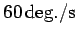, what is its rotational kinetic energy?

Answer: The moment of inertia of a rod of mass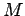and length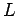about an axis, perpendicular to its length, which passes through its midpoint is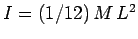. This is a standard result. Using the parallel axis theorem, the moment of inertia about a parallel axis passing through one of the ends of the rod is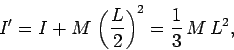so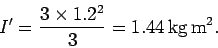The instantaneous angular velocity of the rod is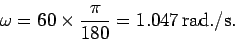Hence, the rod's rotational kinetic energy is written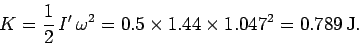Next: Worked example 8.4: Weight Up: Rotational motion Previous: Worked example 8.2: Accelerating
Richard Fitzpatrick 2006-02-02